View Course Path

# VHDL code for flip-flops using behavioral method – full code

Let’s write the VHDL code for flip-flops using behavioral architecture. We will code all the flip-flops, D, SR, JK, and T, using the behavioral modeling method of VHDL. These will be the first sequential circuits that we code in this course on VHDL. We’ll also write the testbenches and generate the final RTL schematics and simulation waveforms for each flip-flop.

Flip-flops are arguably the most important building block of our modern digital electronics. They are memory cells. Note that flip-flops are not to be confused with latches. This is a common confusion. There are certain differences between flip-flops and latches. In fact, flip-flops are built using latches.

Contents

## D flip-flop

### Circuit diagram explanation

The circuit above shows a D flip-flop using an SR latch. The D flip-flop has one input and two outputs. The outputs are complementary to each other. The D in D flip-flop stands for Data or Delay.

Regardless, the circuit’s structural aspect is only necessary to figure out the I/O ports. In behavioral architecture, what’s necessary is the behavior of the circuit. The way the circuit responds to a certain set of inputs. This is given by the truth table.

Truth table for D flip-flop

 CLK D Q Q’ 0 x No change No change 1 0 0 1 1 1 1 0

Let’s dive right into the code. We will be using if-elsif statements as we did in the VHDL code for demultiplexers post. The entity-architecture pair will declare the I/O ports, their datatype, and the architecture of the program. The flip-flop has a Clock input, a reset input, a normal input, and two outputs. We will use the `STD_LOGIC` datatype because these I/Os are separate from each other. Begin the architecture.

### Explanation of the VHDL code

```library IEEE;
use IEEE.STD_LOGIC_1164.ALL;
use IEEE.STD_LOGIC_ARITH.ALL;
use IEEE.STD_LOGIC_UNSIGNED.ALL;

entity D_FLIPFLOP_SOURCE is
Port ( D, CLK, RST : in  STD_LOGIC;
Q, Qb : out  STD_LOGIC);
end D_FLIPFLOP_SOURCE;

architecture Behavioral of D_FLIPFLOP_SOURCE is

begin
```

The `process` statement has a longer sensitivity list than we saw in our previous posts. There are three signals that the process is sensitive to. And that’s fair. Because, from the truth table, a change in the values of any of these signals causes a change in the output. It’s a part of the circuit’s behavior. To encode this feature in the program, we have to then, naturally, include the signals in the process. As the process executes when its sensitivity list is triggered. Begin the process.

```process (D, CLK, RST)
begin
```

If the reset signal is high, the flip-flop just resets. The output will be zero.

```if (RST = '1') then
Q <= '0';
```

Now that we are done with the reset part let’s talk about when the reset is inactive. A D flip-flop made using SR has a positive edge-triggered clock. And it is known as a data flip-flop. However, in a D flip-flop made using JK, the clock is negative edge-triggered. In this case, the flip-flop is known as a Delay flip-flop. Here we will deal with the former. If the clock has a rising edge, then the output Q will be equal to the input. Qb will be complementary to the input.

```elsif (rising_edge(CLK)) then
Q <= D;
Qb <= not D;
```

Remember to close the process, architecture and the if statements. You know how to.

### Complete VHDL code for D flip-flop using behavioral method

```library IEEE;
use IEEE.STD_LOGIC_1164.ALL;
use IEEE.STD_LOGIC_ARITH.ALL;
use IEEE.STD_LOGIC_UNSIGNED.ALL;

entity D_FLIPFLOP_SOURCE is
Port ( D, CLK, RST : in  STD_LOGIC;
Q, Qb : out  STD_LOGIC);
end D_FLIPFLOP_SOURCE;

architecture Behavioral of D_FLIPFLOP_SOURCE is

begin
process (D, CLK, RST)
begin

if (RST = '1') then
Q <= '0';
elsif (rising_edge(CLK)) then ---this is for data flip-flop, for delay flip-flop use negative edge
Q <= D;
Qb <= not D;

end if;
end process;
end Behavioral;
```

### Testbench in VHDL

```library IEEE;
use IEEE.STD_LOGIC_1164.ALL;
use IEEE.STD_LOGIC_ARITH.ALL;
use IEEE.STD_LOGIC_UNSIGNED.ALL;

entity DFF_tb is
end entity;

architecture tb of DFF_tb is

component D_FLIPFLOP_SOURCE is
Port ( D, CLK, RST : in STD_LOGIC;
Q, Qb : out STD_LOGIC);
end component ;

signal D, CLK, RST, Q, Qb : STD_LOGIC;

begin
uut: D_FLIPFLOP_SOURCE port map(
D => D,
CLK => CLK,
RST => RST,
Q => Q,
Qb => Qb);

Clock : process
begin
CLK <= '0';
wait for 10 ns;
CLK <= '1';
wait for 10 ns;
end process;

stim : process
begin

RST <= '0';
D <= '0';
wait for 40 ns;
D <= '1';
wait for 40 ns;

end process;
end tb;```

### RTL Schematic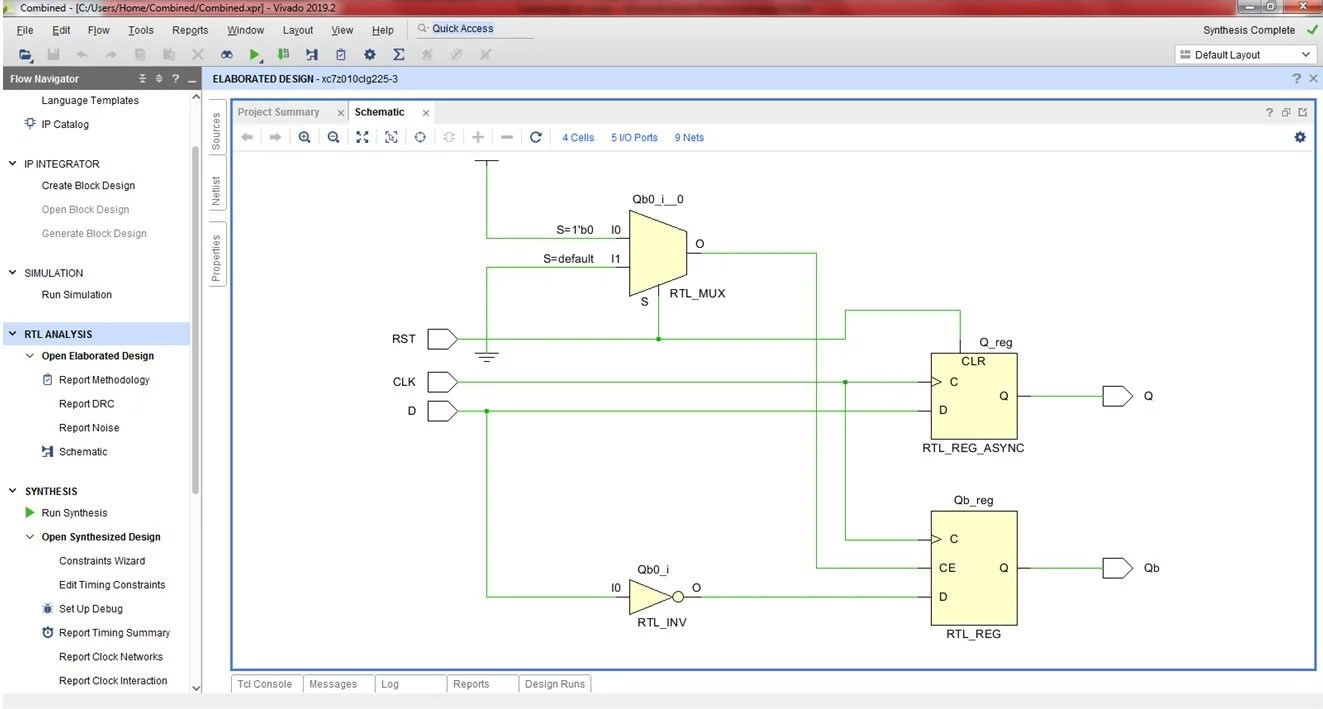### Simulation WaveformNext up, we will code the SR flip-flop in VHDL.

## SR flip-flop

### Circuit diagram explanation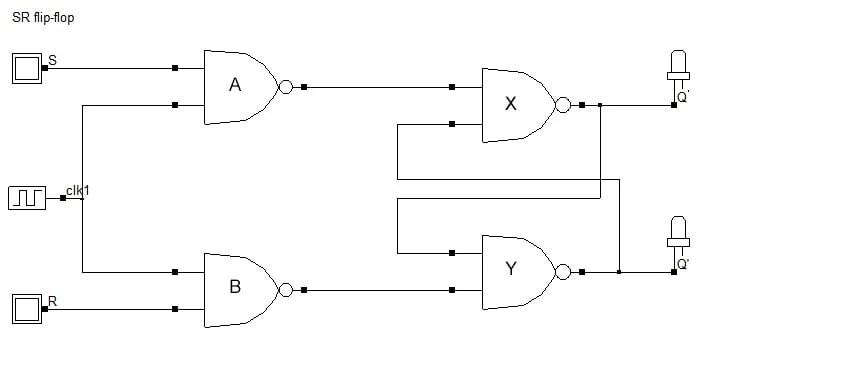The circuit above shows an SR flip-flop with two inputs and two outputs. The outputs are complementary to each other. The SR in SR flip-flop stands for Set-Reset.

Truth table for SR flip-flop

 CLK S R Q Q’ 0 x x Qprv Q’prv 1 0 0 Qprv Q’prv 1 0 1 0 1 1 1 0 1 0 1 1 1 – –

### Explanation of the VHDL code

The SR flip-flop has four `STD_LOGIC` inputs. The reset signal, the clock, and the SR inputs. In addition to that, it also has two `STD_LOGIC` outputs, Q and Qb. Since we are using the behavior modeling style, we have a `process` statement too. The flip-flop’s behavior gets affected by all the input signals. Hence all the input signals make the sensitivity list. Let’s begin.

```library IEEE;
use IEEE.STD_LOGIC_1164.ALL;
use IEEE.STD_LOGIC_ARITH.ALL;
use IEEE.STD_LOGIC_UNSIGNED.ALL;

entity SR_FLIPFLOP_SOURCE is
Port ( S,R,RST,CLK : in  STD_LOGIC;
Q,Qb : out STD_LOGIC);
end SR_FLIPFLOP_SOURCE;

architecture Behavioral of SR_FLIPFLOP_SOURCE is

begin

process (S,R,RST,CLK)

begin
```

Naturally, when the reset signal is active, the output will be 0. When the reset signal is inactive, and a rising edge of the clock is present, the behavior shown in the truth table will be activated. Case 1: if S is not equal to R, then the outputs will mirror S. In the last case, the output has a high impedance. This is denoted by the letter Z in VHDL.

```if (RST = '1') then
Q <= '0';
elsif (RISING_EDGE(CLK))then
if (S /= R) then
Q <= S;
Qb <= R;
elsif (S = '1' AND R = '1') then
Q <= 'Z';
Qb <= 'Z';
```

In the end, we close off the process, the if statements, and the architecture.

```end if;
end if;
end process;
end Behavioral;
```

### Complete VHDL code for SR flip-flop using the behavioral method

```library IEEE;
use IEEE.STD_LOGIC_1164.ALL;
use IEEE.STD_LOGIC_ARITH.ALL;
use IEEE.STD_LOGIC_UNSIGNED.ALL;
entity SR_FLIPFLOP_SOURCE is
Port ( S,R,RST,CLK : in  STD_LOGIC;
Q,Qb : out STD_LOGIC);
end SR_FLIPFLOP_SOURCE;

architecture Behavioral of SR_FLIPFLOP_SOURCE is

begin

process (S,R,RST,CLK)

begin

if (RST = '1') then
Q <= '0';
elsif (RISING_EDGE(CLK))then
if (S /= R) then
Q <= S;
Qb <= R;
elsif (S = '1' AND R = '1') then
Q <= 'Z';
Qb <= 'Z';

end if;
end if;
end process;
end Behavioral;```

### Testbench in VHDL

```library IEEE;
use IEEE.STD_LOGIC_1164.ALL;
use IEEE.STD_LOGIC_ARITH.ALL;
use IEEE.STD_LOGIC_UNSIGNED.ALL;

entity SR_FF_tb is
end entity;

architecture tb of SR_FF_tb is

component SR_FLIPFLOP_SOURCE is
Port ( S,R,RST,CLK : in STD_LOGIC;
Q,Qb : out STD_LOGIC);
end component;

signal S, R, RST, CLK, Q, Qb : STD_LOGIC;

begin
uut: SR_FLIPFLOP_SOURCE port map(
S => S,
R => R,
RST => RST,
CLK => CLK,
Q => Q,
Qb => Qb);

Clock : process
begin

CLK <= '0';
wait for 10 ns;
CLK <= '1';
wait for 10 ns;

end process;

Stim : process
begin

RST <= '0';

S <= '0';
R <= '0';
wait for 20 ns;

S <= '0';
R <= '1';
wait for 20 ns;

S <= '1';
R <= '0';
wait for 20 ns;

S <= '1';
R <= '1';
wait for 20 ns;

end process;
end tb;```

### RTL Schematic### Simulation Waveform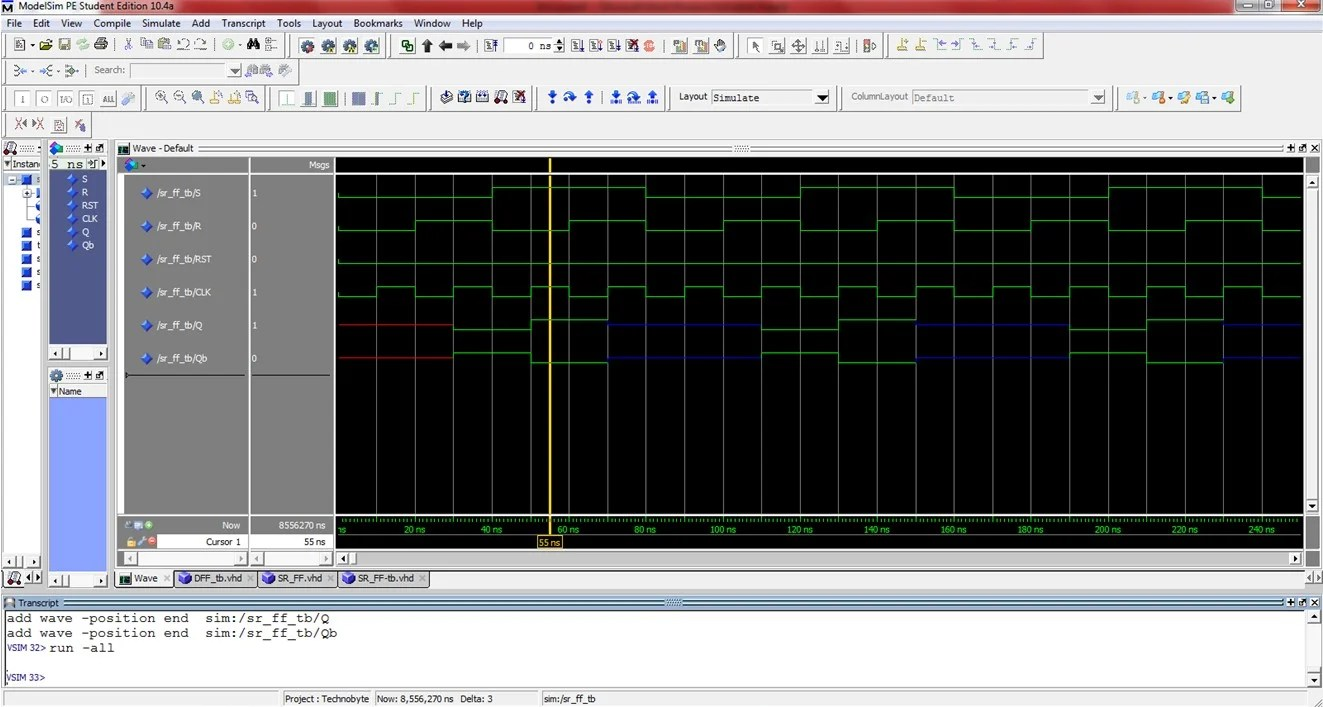## JK flip-flop

### Circuit diagram explanation

The JK flip-flop removes the not allowed condition that occurs when both inputs are high in an SR flip-flop. Additionally, the Master-Slave configuration of the JK flip-flop also removes the race-around-condition.

The race around condition is when a normal JK flip-flop gets stuck in a toggling loop for every clock pulse change when both the inputs are high. The Master-slave configuration ends that loop and stabilizes the output.

Truth table for JK flip-flop

 CLK J K Q Q’ 1 0 0 Qprv Qprv’ 1 0 1 0 1 1 1 0 1 0 1 1 1 Qprv’ Qprv

As you can see, when both the inputs are high, the output is a complement of the previous output.

### Explanation of the VHDL code

Let’s declare the entity-architecture pair first and foremost. There are four input signals. J and K, quite naturally. In addition to that, we have the Clock and the reset inputs too. Q, Qb, and temp are declared as input and output signals using ‘inout’. This is because when the Q signal is assigned to temp, we are using Q as an input. If we declare these as just simple outputs, we will get an error. `STD_LOGIC` datatype shall be used. Since the signals are discrete.

```library IEEE;
use IEEE.STD_LOGIC_1164.ALL;
use IEEE.STD_LOGIC_ARITH.ALL;
use IEEE.STD_LOGIC_UNSIGNED.ALL;
entity JK_FF is
port( J, K, clk, rst : in std_logic;
Q, Qbar : out std_logic);
end JK_FF;

architecture behavioral of JK_FF is

begin```

The process sensitivity list has all the inputs. Let’s begin the process.

```process (clk,rst)
variable qn : std_logic;
begin
```

As with the D and SR flip-flops above, let’s get the reset=high case out of the way using a simple if statement. Then we can start with the case where both the inputs are unequal.

In this case, the output is equal to the J input. In the case where both the inputs are high, we first assign the output to a temp variable. Then the final output is a complement of the temp variable.

The temp variable was just used as a placeholder to allow us the ability to complement the output.

```if(rst = '1')then
qn := '0';
elsif(clk'event and clk = '1')then
if(J='0' and K='0')then
qn := qn;
elsif(J='0' and K='1')then
qn := '0';
elsif(J='1' and K='0')then
qn := '1';
elsif(J='1' and K='1')then
qn := not qn;
else
null;
end if;
else
null;
end if;
Q <= qn;
Qbar <= not qn;```

Last but not least are the closing statements.

### Complete VHDL code for JK flip-flop using behavioral modeling method

```library IEEE;
use IEEE.STD_LOGIC_1164.ALL;
use IEEE.STD_LOGIC_ARITH.ALL;
use IEEE.STD_LOGIC_UNSIGNED.ALL;

entity JK_FF is
port( J, K, clk, rst : in std_logic;
Q, Qbar : out std_logic);
end JK_FF;

architecture behavioral of JK_FF is
begin
process(clk, rst)
variable qn : std_logic;
begin
if(rst = '1')then
qn := '0';
elsif(clk'event and clk = '1')then
if(J='0' and K='0')then
qn := qn;
elsif(J='0' and K='1')then
qn := '0';
elsif(J='1' and K='0')then
qn := '1';
elsif(J='1' and K='1')then
qn := not qn;
else
null;
end if;
else
null;
end if;
Q <= qn;
Qbar <= not qn;

end process;
end behavioral;```

### Testbench in VHDL

```library ieee;
use ieee.std_logic_1164.all;

entity JK_FF_tb is
end JK_FF_tb;

architecture testbench of JK_FF_tb is

component JK_FF is
port(J, K, clk, rst : in std_logic;
Q, Qbar : out std_logic
);
end component;

signal J, K, clk, rst : std_logic;
signal Q, Qbar : std_logic;

begin
uut: JK_FF port map(
J => J,
K => K,
clk => clk,
rst => rst,
Q => Q,
Qbar => Qbar);

clock: process
begin
clk <= '1';
wait for 10 ns;
clk <= '0';
wait for 10 ns;
end process;

Force: process
begin
J <= '0';
K <= '0';
rst <= '0';
wait for 20 ns;

J <= '0';
K <= '1';
rst <= '0';
wait for 20 ns;

J <= '1';
K <= '0';
rst <= '0';
wait for 20 ns;

J <= '1';
K <= '1';
rst <= '0';
wait for 20 ns;

J <= '1';
K <= '1';
rst <= '0';
wait for 20 ns;

J <= '0';
K <= '0';
rst <= '0';
wait for 20 ns;

J <= '0';
K <= '0';
rst <= '1';
wait for 20 ns;
end process;
end testbench;```

### RTL Schematic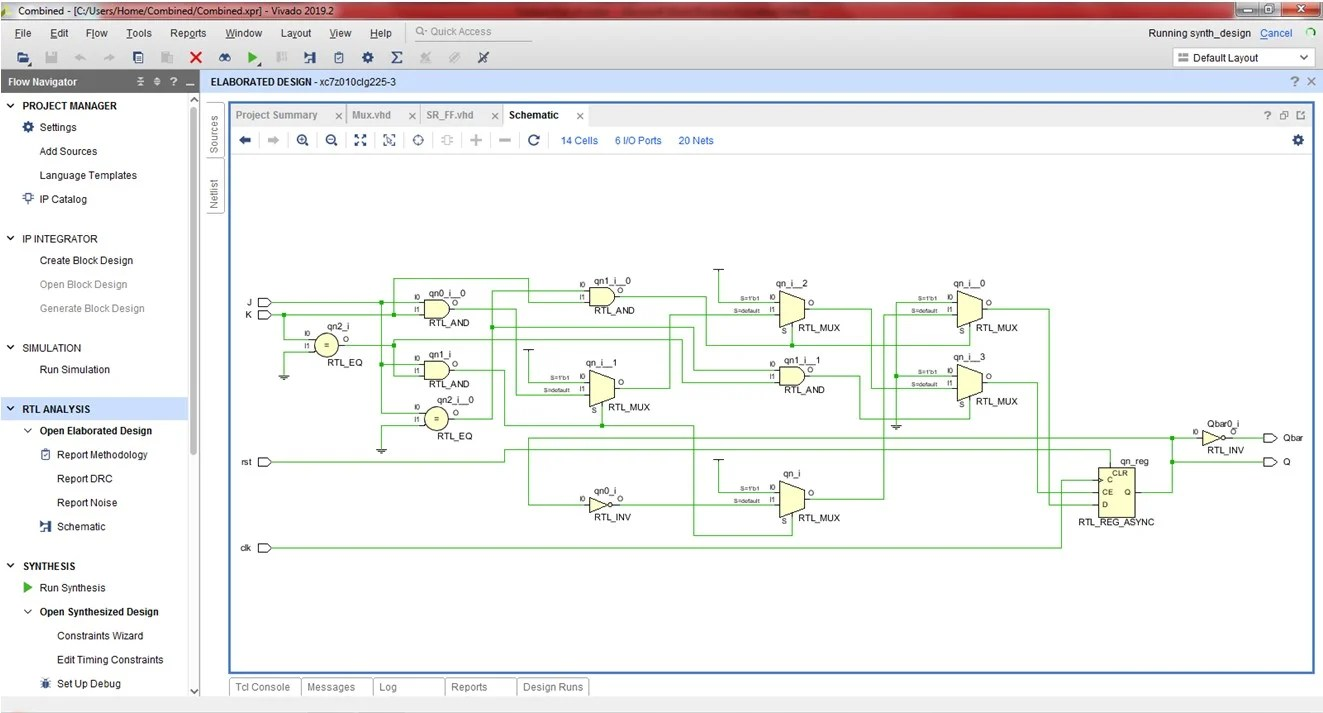### Simulation WaveformsFinally, we will take a look at implementing the VHDL code for T flip-flop using behavioral architecture.

## T flip-flop

### Circuit diagram explanation

The T in T flip-flop stands for ‘toggle’. This is because a T flip-flop toggles (changes) its value whenever the input is high. When the input is low, the output remains the same as the previous output. A T flip-flop can be made using an SR latch, as shown above. Or it can be made using a JK flip-flop as shown below.

### Truth table for T flip-flop

 CLK T Q Q’ 0 x No change No change 1 0 Qprv Qprv’ 1 1 Qprv’ Qprv

The entity will declare the input and output ports for the T flip-flop. We have the clock, the reset, and the T input as actual inputs. The outputs are just the usual Q and Qb. As has been the case with all the remaining flip-flops, we will use behavioral architecture. That’s the entity-architecture pair sorted right there.

```library IEEE;
use IEEE.STD_LOGIC_1164.ALL;
use IEEE.STD_LOGIC_ARITH.ALL;
use IEEE.STD_LOGIC_UNSIGNED.ALL;

entity T_FLIPFLOP_SOURCE is
Port ( T,CLK,RES : in  STD_LOGIC;
Q,QB : out STD_LOGIC);
end T_FLIPFLOP_SOURCE;

architecture Behavioral of T_FLIPFLOP_SOURCE is

begin
```

We will initialize a temp variable within the process too. And then we begin the process.

```PROCESS(T,CLK,RES)
VARIABLE TEMP:STD_LOGIC:='0';
BEGIN
```

The `:=` operator is used for variable assignment in VHDL. The  `<=` or `=>` signs are used for signal assignment in VHDL. However, these rules are not stringent. Since `temp` is a variable here we will use the `:=` operator to assign it a value of 0. However, after the if statements, Q and Qb are signals, and hence we use the signal assignment operator.

```IF(RES='1')THEN
TEMP:='0';
ELSIF(RISING_EDGE(CLK))THEN
IF(T='1')THEN
TEMP:= NOT TEMP;

END IF;
END IF;
Q<= NOT TEMP;
QB<= TEMP;```

Do not forget the closing statements.

### Complete VHDL code for T flip-flop using the behavioral method

```library IEEE;
use IEEE.STD_LOGIC_1164.ALL;
use IEEE.STD_LOGIC_ARITH.ALL;
use IEEE.STD_LOGIC_UNSIGNED.ALL;

entity T_FLIPFLOP_SOURCE is
Port ( T,CLK,RES,TEMP : in  STD_LOGIC;
Q,QB : out STD_LOGIC);
end T_FLIPFLOP_SOURCE;

architecture Behavioral of T_FLIPFLOP_SOURCE is

begin

PROCESS(T,CLK,RES)

VARIABLE TEMP:STD_LOGIC:='0';

BEGIN

IF(RES='1')THEN
TEMP:='0';
ELSIF(RISING_EDGE(CLK))THEN
IF(T='1')THEN
TEMP:= NOT TEMP;

END IF;
END IF;
Q<= NOT TEMP;
QB<= TEMP;

END PROCESS;
END BEHAVIORAL;
```

### Testbench in VHDL

```library IEEE;
use IEEE.STD_LOGIC_1164.ALL;
use IEEE.STD_LOGIC_ARITH.ALL;
use IEEE.STD_LOGIC_UNSIGNED.ALL;

entity TFF_tb is
end entity;

architecture tb of TFF_tb is
component T_FLIPFLOP_SOURCE is
Port ( T,CLK,RES : in STD_LOGIC;
Q,QB : out STD_LOGIC);
end component;

signal T,CLK,RES,Q,QB : STD_LOGIC;

begin
uut: T_FLIPFLOP_SOURCE port map(
T => T,
CLK => CLK,
RES => RES,
Q => Q,
QB => QB);

clock : process
begin

CLK <= '0';
wait for 10 ns;
CLK <= '1';
wait for 10 ns;

end process;

stim: process
begin

RES <= '0';

T <= '0';
wait for 20 ns;

T <= '1';
wait for 20 ns;

end process;
end tb;```

### RTL Schematic### Simulation Waveforms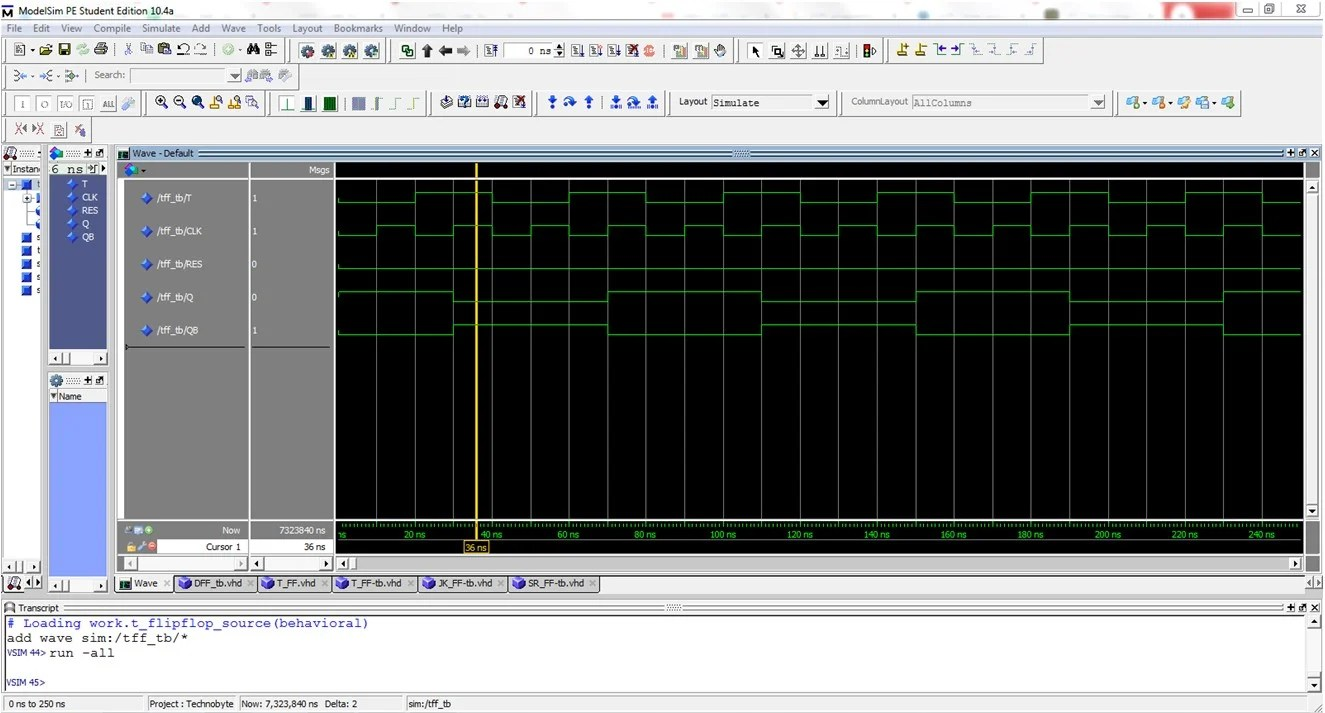Edit: Post updated with the testbench, RTL Schematic, and Simulation Waveform by Deepak Joshi.

This site uses Akismet to reduce spam. Learn how your comment data is processed.

Top